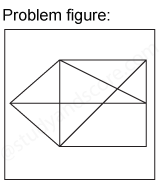## Practice Test Discussion

Q)

In each of the following question, find the minimum number of triangles in the given figure.• ExplanationAfter labelling the figure, we get the following,

1. Simplest triangles ABF, BIC, CIH, GIH, FGE and AFE (6)
2. Triangles with two components each ABE, AGE, BHF, BCH, CGH and BIE (6)
3. Triangles with three components each ABH, BCE and CDE (3)

Total number of triangles in the figure is 6 + 6 + 3 = 15

Hence option D is correct.

- Share with your friends! -